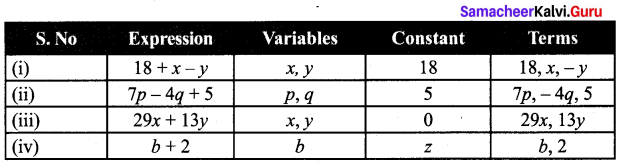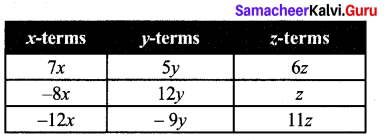Students can Download Maths Chapter 3 Algebra Ex 3.1 Questions and Answers, Notes Pdf, Samacheer Kalvi 7th Maths Book Solutions Guide Pdf helps you to revise the complete Tamilnadu State Board New Syllabus and score more marks in your examinations.

## Tamilnadu Samacheer Kalvi 7th Maths Solutions Term 1 Chapter 3 Algebra Ex 3.1

Question 1.
Fill in the blanks
(i) The variable in the expression 16x – 7 is _____
(ii) The constant term of the expression 2y – 6 is _____
(iii) In the expression 25m + 14M, the type of the terms are ______ terms
(iv) The number of terms in the expression 3ab + 4c – 9 is _____
Hint: Terms are 3ab, 4c – 9.
(v) The numerical co-efficient of the term -xy is ______
Hint: -x,y = (- 1 )xy.
Solution:
(i) x
(ii) -6
(iii) unlike
(iv) three
(v) -1

Question 2.
Say true or False
(i) x + (-x) = 0.
(ii) The co-efficient of ab in the term 15 abc is 15.
Hint: Coefficient of ab is 15c
(iii) 2pq and – 7qp are like terms.
(iv) When y = -1, the value of the expression 2y – 1 is 3.
Hint: 2(-1) – 1 = -2 – 1 = – 3
Solution:
(i) True
(ii) False
(iii) True
(iv) False

Question 3.
Fing the numerical co-efficient of each of the following terms: -3yx, 12k, y, 121bc, -x, 9pq, 2ab.
Solution:
(i) Numerical co-efficient of-3yx is – 3
(ii) Numerical co-efficient of 12k is 12
(iii) Numerical coefficient of y is 1
(iv) Numerical co-efficient of 1216c is 121
(v) Numerical co-efficient of – x is – 1
(vi) Numerical co-efficient of 9pq is 9
(vii) Numerical co-efficient of 2ab is 2Question 4.
Write the variables, constants and terms of the following expressions,
(i) 18 + x – y
(ii) 7p – 4q + 5
(iii) 29x + 13y
(iv) b + 2
Solution:Question 5.
Identify the like terms among the following 7x, 5y, -8x, 12y, 6z, z, -12x, -9y, 11 z
Solution:Question 6.
If x = 2 andy = 3, then find the value of the following expressions,
(i) 2x – 3y
(ii) x + y
(iii) 4y – x
(iv) x + 1 – y
Solution:
Given x = 2; y = 3.
(i) 2x – 3y = 2 (2) – 3 (3) = 4 – 9
= 4 + (Additive inverse of 9)
= 4 +(-9) = -5
(ii) x + y = 2 + 3 = 5
(iii) 4y – x = 4 (3) – 2 = 12 – 2 = 10
(iv) x + 1 – y = 2 + 1 – 3 = 3 – 3 = 0

Objective Type Questions

Question 1.
An algebraic statement which is equivalent to the verbal statement “Three times the sum of ‘x’ and ‘y’ is
(i) 3 (x + y)
(ii) 3 + x + y
(iii) 3x + y
(iv) 3 + xy
Solution:
(i) 3 [(x + y)]

Question 2.
The numerical co-efficient of -7mn is
(i) 7
(ii) -7
(iii) p
(iv) -p
Solution:
(ii) -7

Question 3.
Choose the pair of like terms
(i) 7p, 7x
(ii) 7r, 7x
(iii) – 4x, 4
(iv) – 4x, 7x
Solution:
(iv) -4x, 7xQuestion 4.
The value of 7a – 4b when a = 3, b = 2 is
(i) 21
(ii) 13
(iii) 8
(iv) 32
Solution:
(ii) 13
Hint: 7(3) – 4(2) = 21 – 8 = 13